# Magnetic reluctance facts for kids

Kids Encyclopedia Facts
Magnetic Circuits

Conventional Magnetic Circuits
• Magnetomotive force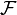$\mathcal F$
• Magnetic flux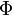$\Phi$
• Magnetic reluctance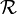$\mathcal R$

Phasor Magnetic Circuits
• Complex reluctance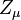$Z_\mu$

Related Concepts
• Magnetic permeability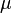$\mu$

Gyrator-capacitor model variables
• Magnetic impedance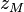$z_M$
• Effective resistance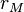$r_M$
• Magnetic inductivity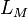$L_M$
• Magnetic capacitivity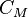$C_M$

Magnetic reluctance, or magnetic resistance, is a measurement used in the analysis of magnetic circuits. It is like resistance in an electrical circuit, but rather than dissipating magnetic energy it stores magnetic energy. As an electric field causes an electric current to follow the path of least resistance, a magnetic field causes magnetic flux to follow the path of least magnetic reluctance. It is a scalar, extensive quantity, like electrical resistance.

Reluctance is usually represented by ℜ.

## History

The term was coined in May 1888 by Oliver Heaviside. The notion of “magnetic resistance” was first mentioned by James Joule and the term "magnetomotive force” (MMF) was first named by Bosanquet. The idea for a magnetic flux law, similar to Ohm's law for closed electric circuits, is attributed to H. Rowland.

## Definition

The total reluctance is equal to the ratio of the "magnetomotive force” (MMF) in a passive magnetic circuit to the magnetic flux in this circuit. In an AC field, the reluctance is the ratio of the amplitude values for a sinusoidal MMF and magnetic flux. (see phasors)

The definition can be expressed as: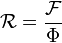$\mathcal R = \frac{\mathcal F}{\Phi}$

where$\mathcal R$ ("R") is the reluctance in ampere-turns per weber (a unit that is equivalent to turns per henry). "Turns" refers to the winding number of an electrical conductor comprising an inductor.$\mathcal F$ ("F") is the magnetomotive force (MMF) in ampere-turns
Φ ("Phi") is the magnetic flux in webers.

It is sometimes known as Hopkinson's law and is analogous to Ohm's Law with resistance replaced by reluctance, voltage by MMF and current by magnetic flux.

Magnetic flux always forms a closed loop, as described by Maxwell's equations, but the path of the loop depends on the reluctance of the surrounding materials. It is concentrated around the path of least reluctance. Air and vacuum have high reluctance. Easily magnetized materials such as soft iron have low reluctance. The concentration of flux in low-reluctance materials forms strong temporary poles and causes mechanical forces that tend to move the materials towards regions of higher flux so it is always an attractive force (pull).

The reluctance of a uniform magnetic circuit can be calculated as: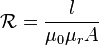$\mathcal R = \frac{l}{\mu_0 \mu_r A}$

or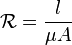$\mathcal R = \frac{l}{\mu A}$

where

l is the length of the circuit in metres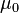$\mu_0$ is the permeability of free space, equal to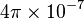$4 \pi \times 10^{-7}$ henry per metre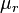$\mu_r$ is the relative magnetic permeability of the material (dimensionless)$\mu$ is the permeability of the material (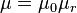$\mu = \mu_0 \mu_r$)
A is the cross-sectional area of the circuit in square metres

The inverse of reluctance is called permeance.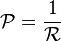$\mathcal P = \frac{1}{\mathcal R}$

Its SI derived unit is the henry (the same as the unit of inductance, although the two concepts are distinct).

## Applications

• Air gaps can be created in the cores of certain transformers to reduce the effects of saturation. This increases the reluctance of the magnetic circuit, and enables it to store more energy before core saturation. This effect is also used in the flyback transformer.
• Variation of reluctance is the principle behind the reluctance motor (or the variable reluctance generator) and the Alexanderson alternator. In other words, the reluctance forces seek the most aligned magnetic circuit and a small air gap distance.
• Multimedia loudspeakers are typically shielded magnetically, in order to reduce magnetic interference they make on televisions and other CRTs. The speaker magnet is covered with a material such as soft iron to minimize the stray magnetic field.

Reluctance can also be applied to:

• Reluctance motors
• Variable reluctance (magnetic) pickups

## Related pages

• Dielectric complex reluctance
• Magnetic capacitivity
• Magnetic capacitance
• Magnetic circuit
• Magnetic complex reluctance
• Reluctance motorMagnetic reluctance Facts for Kids. Kiddle Encyclopedia.